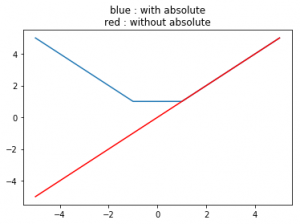# numpy.absolute() in Python

numpy.absolute(arr, out = None, ufunc ‘absolute’) : This mathematical function helps user to calculate absolute value of each element. For complex input, a + ib, the absolute value is.

Parameters :

arr  : [array_like] Input array or object whose elements, we need to test.


Return :

An array with absolute value of each array.


Code #1 : Working

 # Python program explaining  # absolute () function     import numpy as np     arr1 = [1, -3, 15, -466]  print ("Absolute Value of arr1 : \n",                      np.absolute(arr1))     arr2 = [23 , -56]  print ("\nAbsolute Value of arr2 : \n",                          np.absolute(arr2))

Output :

Absolute Value of arr1 :
[  1   3  15 466]

Absolute Value of arr2 :
[23 56]


Code #2 : Working with complex numbers

 # Python program explaining  # absolute () function     import numpy as np     a = 4 + 3j print("Absolute(4 + 3j) : ",               np.absolute(a))     b = 16 + 13j print("\nAbsolute value(16 + 13j) : ",                          np.absolute(b))

Output :

Absolute(4 + 3j) :  5.0

Absolute value(16 + 13j) :  20.6155281281

Code #3: Graphical Representation of numpy.absolute()

 # Python program explaining  # absolute () function     import numpy as np  import matplotlib.pyplot as plt     a = np.linspace(start = -5, stop = 5,                  num = 6, endpoint = True)                     print("Graphical Representation : \n",                          np.absolute(a))     plt.title("blue : with absolute\nred : without absolute")  plt.plot(a, np.absolute(a))     plt.plot(a, a, color = 'red')  plt.show()

Output :

Graphical Representation :
[ 5.  3.  1.  1.  3.  5.]References :
https://docs.scipy.org/doc/numpy-1.13.0/reference/generated/numpy.absolute.html
.

My Personal Notes arrow_drop_upIf you like GeeksforGeeks and would like to contribute, you can also write an article using contribute.geeksforgeeks.org or mail your article to contribute@geeksforgeeks.org. See your article appearing on the GeeksforGeeks main page and help other Geeks.

Please Improve this article if you find anything incorrect by clicking on the "Improve Article" button below.

Article Tags :

Be the First to upvote.

Please write to us at contribute@geeksforgeeks.org to report any issue with the above content.